# NCERT Class 8 Maths Chapter 15 – Introduction to Graphs Solutions

## Exercise 15.1 PageNo: 236

1. The following graph shows the temperature of a patient in a hospital, recorded every hour.

(a) What was the patient’s temperature at 1 p.m.?

(b) When was the patient’s temperature 38.5° C?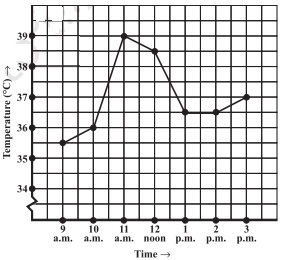(c) The patient’s temperature was the same two times during the period given.

What were these two times?

(d) What was the temperature at 1.30p.m.? How did you arrive at your answer?

(e) During which periods did the patients’ temperature show an upward trend?

Solution:

(a) The patient’s temperature was 36.5oC at 1 p.m.

(b) The patient’s temperature was 38.5oC at 12noon.

(c) The patient’s temperature was same at 1 p.m. and 2p.m

(d)The temperature at 1.30p.m. is 36.5oC.

The point between 1p.m.and 2 p.m., x-axis is equidistant from the two points showing 1p.m. and 2p.m. Soit represents 1.30 p .m similarly the point on y axis, between 36C and 37C will Represent 36.5C.

(e) The patient’s temperature showed an upward trend from 9a.m.to 11a.m. and from 2 p.m. to 3 p.m.

2. The following line graph shows the yearly sales figures for a manufacturing company.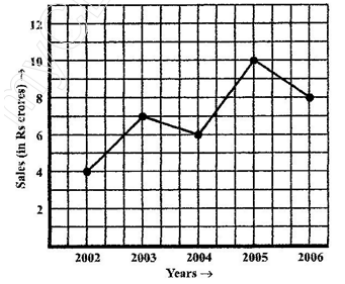(a) What were the sales in (i) 2002 (ii) 2006?

(b) What were the sales in (i) 2003 (ii) 2005?

(c) Compute the difference between the sales in 2002and 2006.

(d) In which year was there the greatest difference between the sales as compared to its

previous year?

Solution:

(a) The sales in:

(i) 2002 was Rs.4 crores and (ii) 2006 was Rs.8 crores

(b) The sales in:

(i) 2003 was Rs.7 crores and (ii) 2005 was Rs.10 crores.

(c) The difference of sales in 2002 and 2006 = Rs.8 crores–Rs.4 crores= Rs.4 crores

(d)In the year 2005, there was the greatest difference between the sales and compared to its previous year, which is (Rs. 10crores –Rs. 6crores) = Rs. 4crores.

3. For an experiment in Botany, two different plants, plant A and plant B were grown under similar laboratory conditions. Their heights were measured at the end of each week for 3 weeks. The results are shown by the following graph.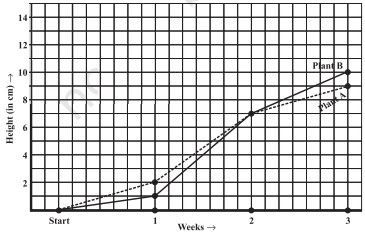(a) How high was Plant A after (i) 2 weeks (ii) 3weeks?

(b) How high was Plant B after (i) 2 weeks (ii) 3 weeks?

(c) How much did Plant A grow during the 3rd week?

(d) How much did Plant B grow from the end of the 2nd week to the end of the 3rd week?

(e) During which week did Plant A grow most?

(f) During which week did Plant B grow least?

(g) Were the two plants of the same height during any week shown here? Specify.

Solution:

(a)

(i)The plant A was 7 cm high after 2 weeks and

(ii) After 3 weeks it was 9 cm high

(b)

(i)Plant B was also 7cm high after 2 weeks and

(ii) After 3 weeks it was 10 cm high

(c) Plant A grew=9 cm–7 cm = 2cm during 3rd week.

(d) Plant B grew during end of the 2nd week to the end of the 3rdweek= 10cm–7cm= 3cm

(e) Plant A grew the highest during second week.

(f)Plant B grew the least during first week.

(g) Yes. At the end of the second week, plant A and B were of the same height, which is 7 cm.

4. The following graph shows the temperature forecast and the actual temperature for each day of a week.

(a) On which days was the forecast temperature the same as the actual temperature?

(b) What was the maximum forecast temperature during the week?

(c) What was the minimum actual temperature during the week?

(d) On which day did the actual temperature differ the most from the forecast temperature?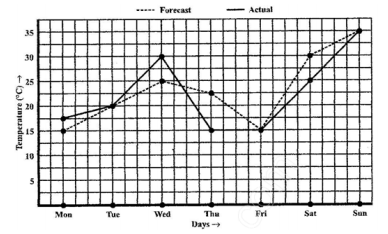Solution:

(a) On Tuesday, Friday and Sunday, the forecast temperature was same as the actual

temperature.

(b) The maximum forecast temperature was 35oC.

(c) The minimum actual temperature was 15oC.

(d) The actual temperature differed the most from the forecast temperature on

Thursday.

5. Use the tables below to draw linear graphs

(a) The number of days a hill side city received snow in different years.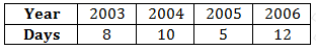(b) Population (in thousands) of men and women in a village in different years.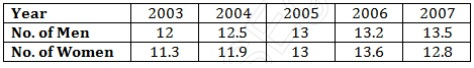Solution:

(a) Consider “Years” along x-axis and “Days” along y-axis. Using given information, linear graph will looks like: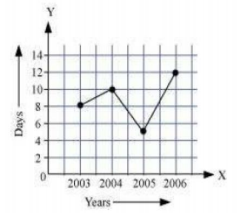(b) Consider “Years” along x-axis and “No. of Men and No. of Women” along y-axis (2 graphs). Using given information, linear graph will looks like: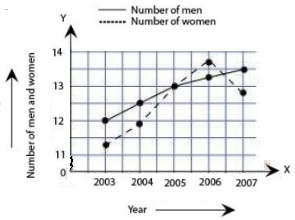6. A courier-person cycles from a town to a neighboring suburban area to deliver a parcel to a merchant. His distance from the town at different times is shown by the following graph.

(a) What is the scale taken for the time axis?

(b) How much time did the person take for the travel?

(c) How far is the place of the merchant from the town?

(d) Did the person stop on his way? Explain.

(e) During which period did he ride fastest?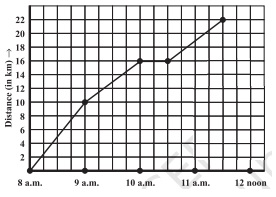Solution:

(a) 4 units = 1hour

(b) The person took 3 ½ hours for the travel.

(c) It was 22 km far from the town.

(d) Yes, this has been indicated by the horizontal part of the graph. He stayed from 10 a.m. to 10.30 a.m.

(e) He rides the fastest between 8 a.m. and 9a.m.

7. Can there be a time-temperature graph as follows? Justify your answer.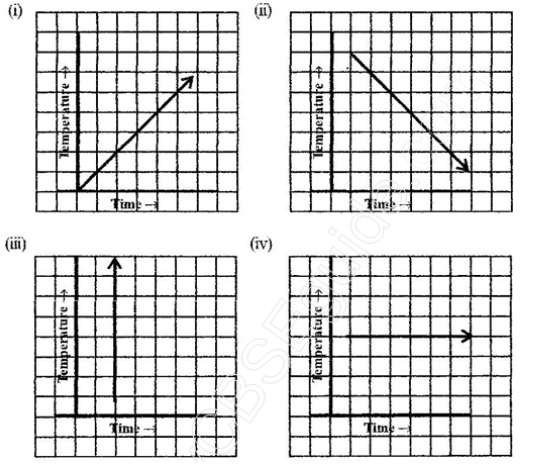Solution:

(i) It is a time-temperature graph. It is showing the increase in temperature as time increases.

(ii) It is a time-temperature graph. It is showing the decrease in temperature as time increases.

(iii) The graph figure (iii)is not possible since temperature is increasing very rapidly

which is not possible.

(iv) It is a time-temperature graph. It is showing constant temperature.

## Exercise 15.2 PageNo: 243

1. Plot the following points on a graph sheet. Verify if they lie on a line

(a) A(4,0), B(4, 2),C(4,6), D(4, 2.5)

(b) P(1, 1), Q(2, 2), R(3,3), S(4, 4)

(c) K(2, 3), L(5, 3), M(5,5), N(2, 5)

Solution:

Plot all the points on the graph.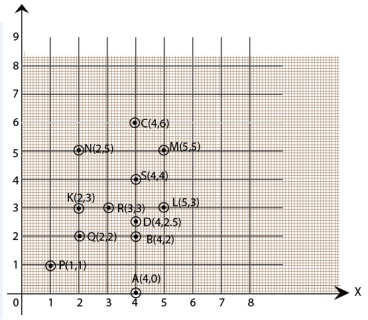(a) All points A,B, C and D lie on a vertical line.

(b) P,Q, R and S points also make a line .It verifies that these points lie on a line.

(c) Points K, L, M and N. These points do not lie in a straight line.

2. Draw the line passing through (2,3)and(3,2).Find the coordinates of the points at which this line meets the x-axis and y-axis.

Solution:

Graph for the Line passes through points (2, 3) and (3, 2) is:The coordinates of the points at which this line meets the x-axis at (5, 0) and

Y axis at (0,5).

3. Write the coordinates of the vertices of each of these adjoining figures.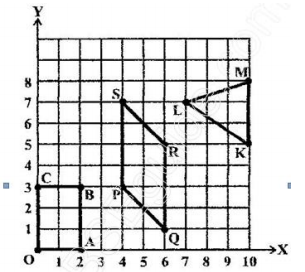Solution:

We can observe three figures named as, OABC, PQRS and LMK.

Vertices of figure OABC

O (0, 0), A (2, 0), B (2, 3) and C (0, 3)

Vertices of figure PQRS

P (4, 3), Q (6, 1), R (6, 5) and S (4, 7)

Vertices of figure LMK

L (7, 7), M(10, 8) and K(10,5)

4. State whether True or False. Correct that are false.

(i) A point whose x coordinate is zero and y-coordinate is non-zero will lie on the y-axis.

(ii) A point whose y coordinate is zero and x-coordinate is 5 will lie on y-axis.

(iii) The co-ordinates of the origin are (0, 0).

Solution:

i) True

ii) False, it will lie on x axis

(iii) True

## Exercise 15.3 Page No: 247

1. Draw the graphs for the following tables of values, with suitable scales on the axes.

(a) Cost of apples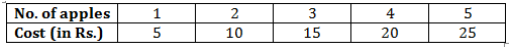(b) Distance travelled by a car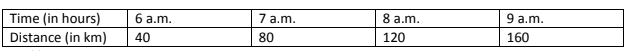(i) How much distance did the car cover during the period 7.30 a.m. to 8 a.m.?

(ii) What was the time when the car had covered a distance of 100 km since it’s start?

(c) Interest on deposits for a year.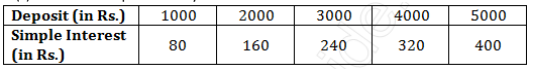(i) Does the graph pass through the origin?

(ii) Use the graph to find the interest on Rs 2500 for a year.

(iii) To get an interest of Rs. 280 per year, how much money should be deposited?

Solution:

Mark “number of apples” on x-axis and “cost” on y-axis. The graph is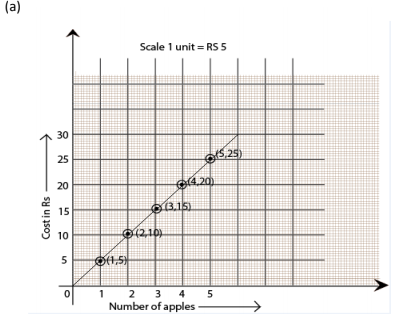(b) Represent the “time” on x-axis and “distance” on y-axis.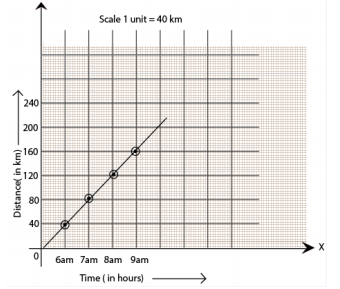(i) The car covered 20 km distance

(ii) It was 7.30 am, when it covered 100 km distance.

(c) Represent “Deposit” on y-axis and “simple interest” on x-axis.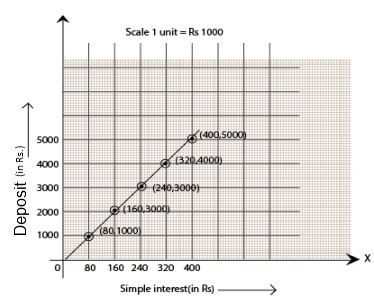(i) Yes, the graph passes through the origin.

(ii) Interest on Rs. 2500 is Rs. 200 for a year.

(iii) Rs. 3500 should be deposited for interest of Rs. 280

2. Draw a graph for the following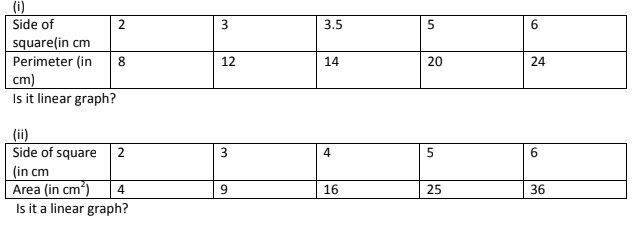Solution:

(i) Yes, it is linear graph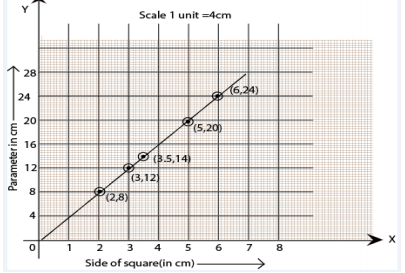(ii) No, it is not a linear graph because the graph does not provide a straight line.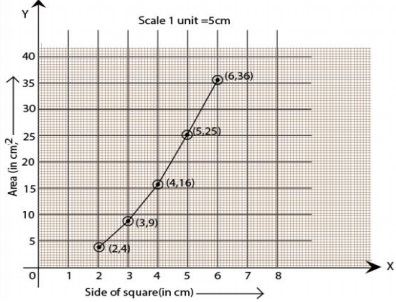Access Class-8 Chapter-wise Solutions: